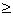Tuesday 29th September 2020CBSE Guess > Papers > Important Questions > Class XII > 2009 > Maths > Mathematics By Anil Kumar Tondak

CBSE CLASS XII

Linear Programming

Q. 1. One kind of cake requires 200gm of flour and 25gm of fat, and another kind of cake requires 100gm of flour and 50gm of fat. Find the maximum number of cakes which can be made from 5 kg of flour and 1kg of fat assuming that there is no shortage of the other ingredients used in making the cakes.

Q. 2. A dietician wishes to mix together two kinds of food X and Y in such a way that the mixture contains at least 10 units of vitamin A, 12 units of vitamin B and 8 units of vitamin C. The Vitamin contents of one Kg food is given below :-

Food X Y Vitamin A Vitamin B Vitamin C 1 2 3 2 2 1

One kg.of food X costs Rs. 16 and one Kg of food Y costs Rs. 20. Find the least cost of the mixture which will produce the required diet.

Q. 3. Maximise and Minimise: Z = x + 2y Subject to constraints x + 2y100 2x – y < 0 2x + y < 200 x, y0

Q. 4. An aeroplane can carry a maximum of 200 passengers. A profit of Rs. 1000 is made on each executive class ticket and a profit of Rs. 600 is made on each economy class ticket. The airline reserves at least 20 seats for executive class. However, at least 4 times as many passengers prefer to travel by economy class than by the executive class. Determine how many tickets of each type must be sold in order to maximise the profit for the airline. What is the maximum profit.

Q. 5. Two godowns A and B have grain capacity of 100 quintals and 50 quintals respectively. They supply to 3 ration shops, D, E and F whose requirements are 60, 50 and 40 quintals respectively. The cost of transportation per quintal from the godowns to the shops are given in following table :

Transportation Cost Per Quintal (in Rs.)

 From / To A B D 6 4 E 3 2 F 2.50 3

How should the supplies be transported in order that the transportation cost is minimum. What is the minimum cost.

Q. 6. Two tailors A and B earn Rs. 150 and Rs. 200 per day respectively. A can stitch 6 shirts and 4 pants per day, while B can stitch 10 shirts and 4 pants per day. Form a linear programming problem to minimize the labour cost to produce at least 60 shirts and 32 pants.

Q. 7. Solve the following L.P.P graphically:
Maximise:    Z = 60x + 15y
Subject to constraints
x + y < 50
3x + y < 90,            x, y0

Q. 8. A dealer wishes to purchase a number of fans and sewing machines. He has only Rs 5,760 to invest and has space for at most 20 items.  A fan costs him Rs. 360 and a sewing machine Rs 240. His expectation is that he can sell a fan at a profit of Rs. 22 and a sewing machine at a profit of Rs. 18. Assuming that he can sell all the items that he can buy, how should he invest his money in order to maximise his profit.

Q. 9. If a young man drives his vehicle at 25 km/hr, he has to spend Rs. 2/km on petrol. If he drives it at a faster speed of 40km/hr, the petrol cost increases to Rs. 5/km. He has Rs. 100 to spend on petrol and travel within one hour. Express this as an L.P.P. and solve.

Prepared By :- Anil Kumar Tondak
M.Sc.(Maths), M.Ed., M.A.(Eco),M.B.A.(Mkt)
Mob No. 9811363962

 Maths